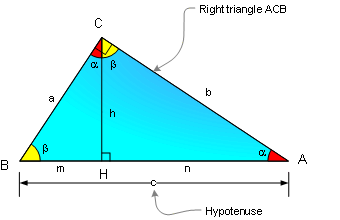# Albert Einstein and the Pythagorean theorem

All,

Here is a neat proof of the Pythagorean theorem that Einstein created when he was 12 years old. Like most of Einstein's discoveries, they are simple in nature and are often a result of simple thought experiment.If we take any right triangle, and we split it down the C vertex, you will end up with three similar triangles.

Similar triangles , are triangles that are proportional to each other. In other words you could ZOOM/SHRINK one and end up with the other. Triangles are similar when their respective angles are the same. Notice the yellow, red, and 90 degree angles are shared amongst the three triangles.  All three triangles have the same interior three angles.

When triangles are similar the are proportional to each other. (keep this in mind)

Please notice that there three triangles in this picture. Triangle A, is the small triangle to the left (with a side). Triangle B, is the small triangle to the right (with b side), and Triangle C which is the sum of triangle A and B.

So Einstein said that the Area of triangle A  is AREAa = a^2 * k .  Please note that the normal area equation that we all know and love is 1/2 *BASE * HEIGHT. So what is this k business?

Einstein said a^2 (a squared) is the area of a square (a by a). The small triangle A would lie within the square(a^2) and be a fraction of that square. So the area of triangle A is a^2 *k.

Hence, the area of triangle B (with the b side) is b^2*k.

Why is this? Well remember the triangles are similar. So, they are all a proportion of each other. If triangle A is k* a^2, then triangle B will be k * b^2. "k" is a number that we don't know and don't care. We could actually solve for it, but it is not needed.

So what do we have so far:  AREAa = a^2 *k ,   AREAb = b^2*k,  AREAc = c^2 *k.

Since the area of triangle A + B must equal to C, then   AREAa + AREAb = AREAc.

Therefore,  (a^2*k) + (b^2*k) = (c^2*k) .

Now divide both sides by the mysterious and magical k, and we have     a^2 + b^2 = c^2.

Ta Da!!! :)

Please note the irony of this solution. Einstein , later went on to prove that E = m * c^2.  :)

(feel free to email me if you have questions.)

This entry was posted in Problems. Bookmark the permalink.